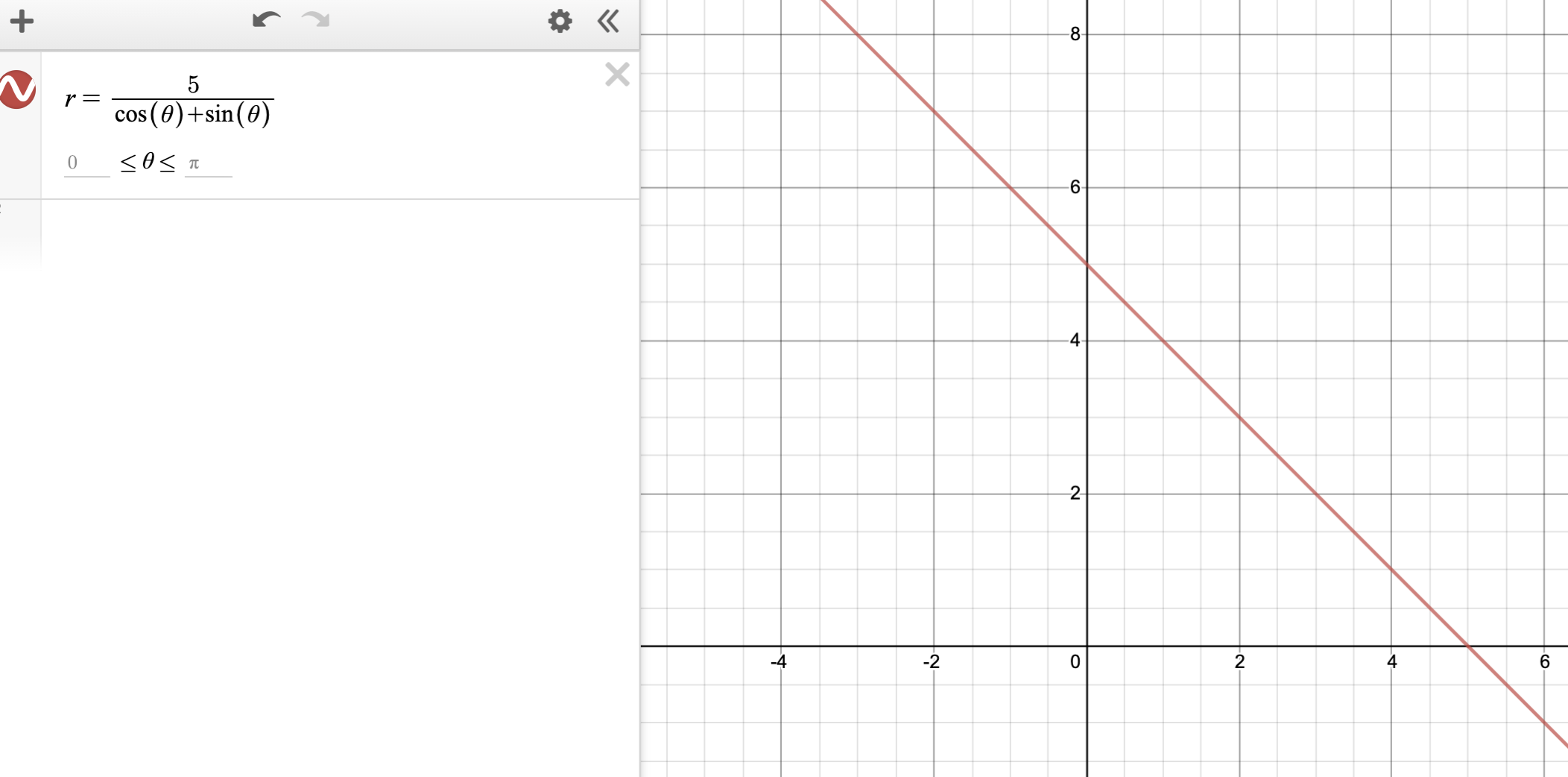### Home > PC3 > Chapter 11 > Lesson 11.1.2 > Problem11-30

11-30.

In this lesson you graphed $r =\frac { 5 } { \ cos ( \theta ) + \sin ( \theta ) }$. If you do not recall the shape of the curve, graph the equation on a calculator.1. Predict the shape of the graph r = $\frac { - 2 } { \operatorname { cos } ( \theta ) + \operatorname { sin } ( \theta ) }$.

2. Check your prediction with a calculator.